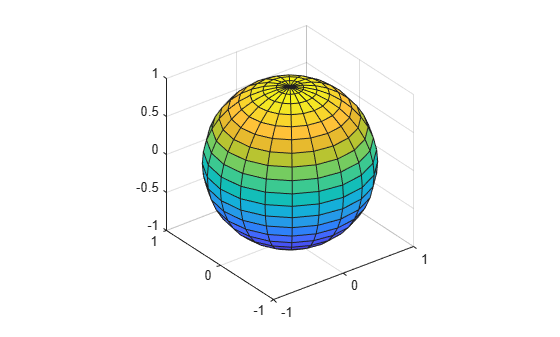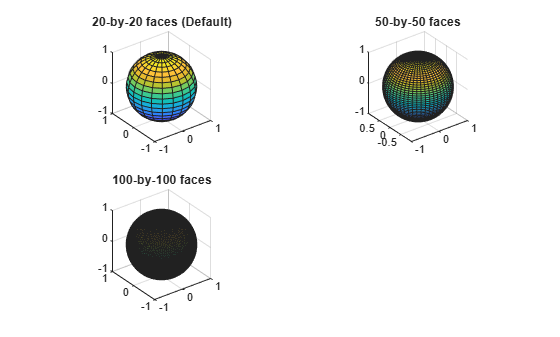sphere

Description

example

[X,Y,Z] = sphere returns the x-, y-, and z- coordinates of a sphere without drawing it. The returned sphere has a radius equal to 1 and consists of 20-by-20 faces.

The function returns the x-, y-, and z- coordinates as three 21-by-21 matrices.

To draw the sphere using the returned coordinates, use the surf or mesh functions.

example

[X,Y,Z] = sphere(n) returns the x-, y-, and z- coordinates of a sphere with a radius equal to 1 and n-by-n faces. The function returns the x-, y-, and z- coordinates as three (n+1)-by-(n+1) matrices.

example

sphere(___) plots the sphere without returning the coordinates. Use this syntax with any of the input arguments in previous syntaxes.

example

sphere(ax,___) plots into the axes specified by ax instead of the current axes. Specify the axes as the first input argument.

Examples

collapse all

Create and plot a sphere with a radius equal to 1. Use axis equal to use equal data units along each coordinate direction.

sphere
axis equalSpecify the radius and location of a sphere by modifying the returned X, Y, and Z coordinates.

Define X, Y, and Z as coordinates of a unit sphere.

[X,Y,Z] = sphere;

Plot the unit sphere centered at the origin.

surf(X,Y,Z)
axis equalDefine X2, Y2, and Z2 as coordinates of a sphere with a radius of 5 by multiplying the coordinates of the unit sphere. Plot the second sphere, centering it at (5,-5,0).

hold on
r = 5;
X2 = X * r;
Y2 = Y * r;
Z2 = Z * r;

surf(X2+5,Y2-5,Z2)Call the tiledlayout function to create a 2-by-2 tiled chart layout. Call the nexttile function to create the axes. Then, use the sphere function to plot three spheres with different numbers of faces into different tiles of the chart by specifying the axes.

tiledlayout(2,2);
ax1 = nexttile;
sphere(ax1);
axis equal
title('20-by-20 faces (Default)')

ax2 = nexttile;
sphere(ax2,50)
axis equal
title('50-by-50 faces')

ax3 = nexttile;
sphere(ax3,100)
axis equal
title('100-by-100 faces')Input Arguments

collapse all

Number of faces, specified as a positive integer.

Target axes, specified as an Axes object. If you do not specify the axes, then sphere plots into the current axes.